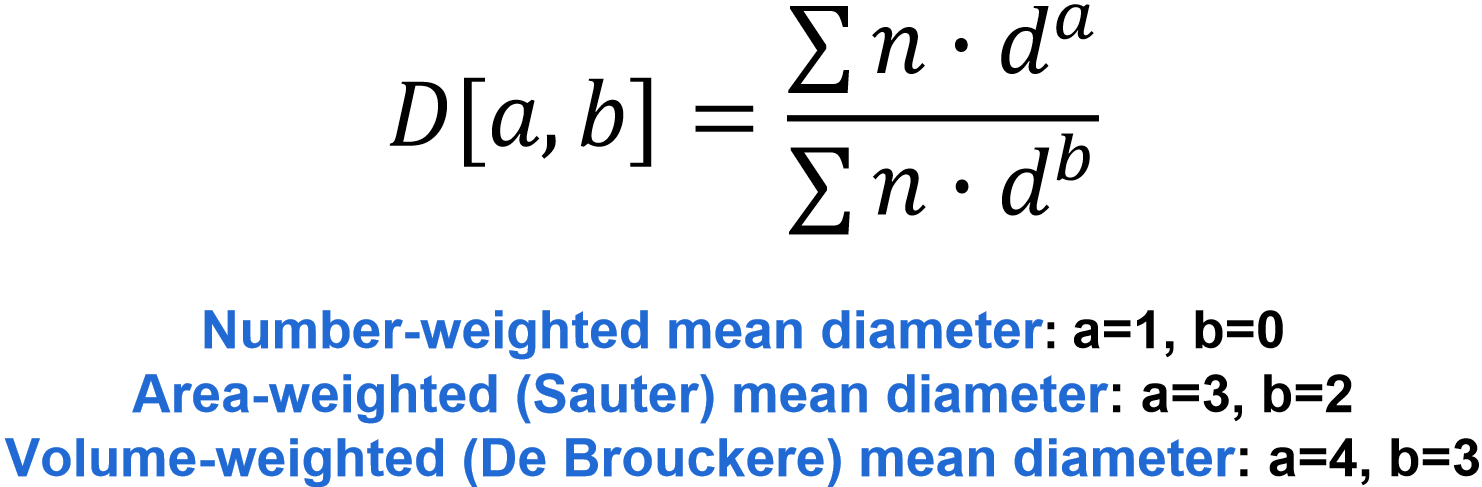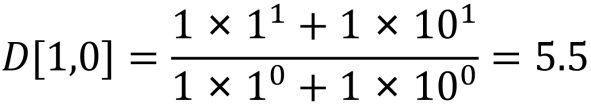Hmoe > Learn > Wiki > What are D[4,3] and D[3,2]?

# What are D[4,3] and D[3,2]?

2023-07-13WIKI

D[4,3] and D[3,2] are the mean diameters of the particle size based on volume-weighted and area-weighted mean results, respectively. Different mean diameters are used to compare results with different techniques. For example, if results from the volume-based laser diffraction method are compared, then the D[4,3] volume-weighted mean result is preferred. If, however, the measurement results emanating from an image analysis system are compared, D[3,2] area-weighted mean result is prioritized.

The calculation of the mean diameter based on different weighting is shown below:For example, two spheres are available, whose diameters are 1μm and 10μm, respectively. If the number-weighted mean diameter is required, then the number-weighted mean diameter is:In this case, the mean diameter shows the quantitative relationship that corresponds to number-weighted.

Suppose the mean diameter of the system needs to be systematically reflected. In that case, the volume-weighted mean diameter shown below should be applied, which indicates that the volume of the large particle has occupied more than 99.9% of the system volume.Struggling to grasp particle analysis concepts? Our new Beginner's Guide to Particle Analysis is here to help you grasp these concepts in the most accessible manner possible. Download it as a PDF to read on the train, on the plane, or wherever else there’s no internet.